# Category: games

At the moment I’m re-reading Siobhan Roberts’ biography of John Horton Conway, Genius at play – the curious mind of John Horton Conway.

In fact, I’m also re-reading Alexander Masters’ biography of Simon Norton, The genius in my basement – the biography of a happy man.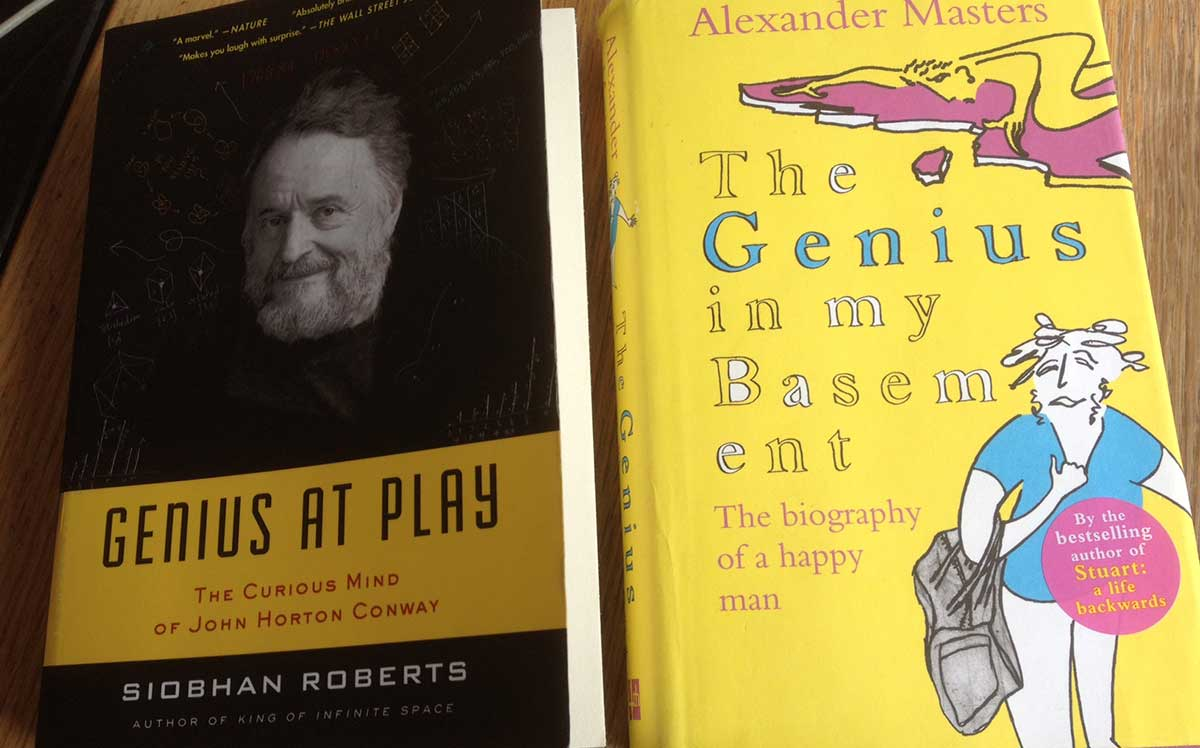If you’re in for a suggestion, try to read these two books at about the same time. I believe it is beneficial to both stories.

Whatever. Sooner rather than later the topic of Conway’s game of life pops up.

Conway’s present pose is to yell whenever possible ‘I hate life!’. Problem seems to be that in book-indices in which his name is mentioned (and he makes a habit of checking them all) it is for his invention of the game of Life, and not for his greatest achievement (ihoo), the discovery of the surreal numbers.

If you have an hour to spare (btw. enjoyable), here are Siobhan Roberts and John Conway, giving a talk at Google: “On His LOVE/HATE Relationship with LIFE”

By synchronicity I encounter the game of life now wherever I look.

Today it materialised in following up on an old post by Richard Green on G+ on Gaussian primes.

As you know the Gaussian integers $\mathbb{Z}[i]$ have unique factorization and its irreducible elements are called Gaussian primes.

The units of $\mathbb{Z}[i]$ are $\{ \pm 1,\pm i \}$, so Gaussian primes appear in $4$- or $8$-tuples having the same distance from the origin, depending on whether a prime number $p$ remains prime in $\mathbb{Z}[i]$ or splits.

Here’s a nice picture of Gaussian primes, taken from Oliver Knill’s paper Some experiments in number theory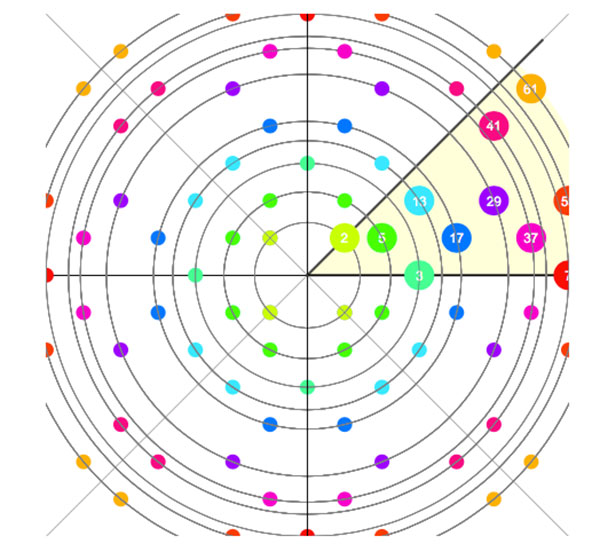Note that the natural order of prime numbers is changed in the process (look at the orbits of $3$ and $5$ (or $13$ and $17$).

Because the lattice of Gaussian integers is rectangular we can look at the locations of all Gaussian primes as the living cell in the starting position on which to apply the rules of Life.

Here’s what happens after one move (left) and after three moves (right):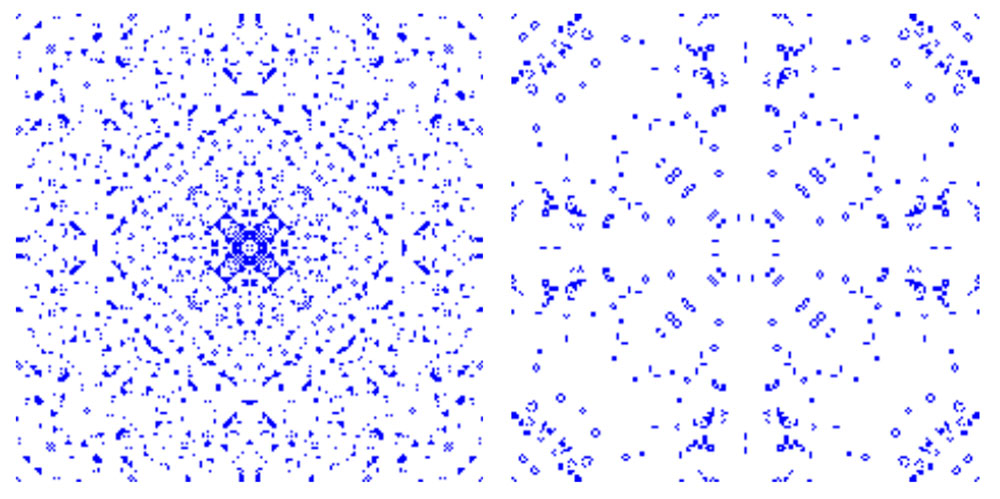Knill has a page where you can watch life on Gaussian primes in action.

Even though the first generations drastically reduce the number of life spots, you will see that there remains enough action, at least close enough to the origin.

Knill has this conjecture:

When applying the game of life cellular automaton to the Gaussian primes, there is motion arbitrary far away from the origin.

What’s the point?

Well, this conjecture is equivalent to the twin prime conjecture for the Gaussian integers $\mathbb{Z}[i]$, which is formulated as

“there are infinitely pairs of Gaussian primes whose Euclidian distance is $\sqrt{2}$.”

Soon, we will be teaching computational geometry courses to football commentators.

If a player is going to be substituted we’ll hear sentences like: “no surprise he’s being replaced, his Voronoi cell has been shrinking since the beginning of the second half!”

David Sumpter, the author of Soccermatics: Mathematical Adventures in the Beautiful Game, wrote a nice article over at Medium The geometry of attacking football.

As an example, he took an attack of Barcelona against Panathinaikos.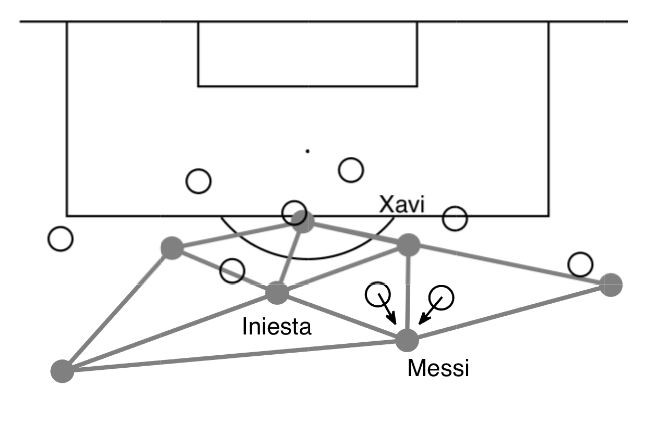and explained the passing possibilities in terms of the Delaunay triangulation between the Barca-players (the corresponding Voronoi cell decomposition is in the header picture).

He concludes: “It is not only their skill on the ball, but also their geometrically accurate positioning that allows them to make the pass.”

Jaime Sampaoi produced a short video of changing Voronoi cells from kick-off by the blue team, with the red team putting pressure until a faulty pass is given, leading to a red-attack and a goal. All in 29 seconds.

I’d love to turn this feature on when watching an actual game.

Oh, and please different cell-colours for the two teams.

And, a remote control to highlight the Voronoi cell of a particular player.

It was fun following the second game last night in real time. Carlsen got a winning endgame with two bishops against a rook, but blundered with 62. Bg4?? (winning was Kf7), resulting in stalemate.

“The computer has just announced that white mates in 31 moves. Of course, the only two people in the building who don’t benefit from that knowledge are behind the pieces.”

[section_title text=”Alice’s game from ‘Through the Looking-Glass'”]

The position below comes from the preface of Lewis Carroll’s Through the Looking-GlassThe old notation for files is used:

a = QR (queen’s side rook)
b = QKt (queen’s side knight)
c = QB (queen’s side bishop)
d = Q (queen)
e = K (king)
f = KB (king’s side bishop)
g = KKt (king’s side knight)
h = KR (king’s side rook)

Further, the row-number depends on whose playing (they both count starting from their own side). Here’s an animated version of the game:And a very strange game it is.

White makes consecutive moves, which is allowed in some versions of fairy chess.

And, as the late Martin Gardner explains in his book The Annotated Alice:

“The most serious violation of chess rules occurs near the end of the
problem, when the White King is placed in check by the Red Queen without
either side taking account of the fact. “Hardly a move has a sane purpose,
from the point of view of chess,” writes Mr. Madan. It is true that both sides
play an exceedingly careless game, but what else could one expect from the
mad creatures behind the mirror? At two points the White Queen passes up
a chance to checkmate and on another occasion she flees from the Red
Knight when she could have captured him. Both oversights, however, are in
keeping with her absent-mindedness.”

In fact, the whole game reflects the book’s story (Alice is the white pawn travelling to the other side of the board), with book-pages associated to the positions listed on the left. Martin Gardner on this:

“Considering the staggering difficulties involved in dovetailing a chess
game with an amusing nonsense fantasy, Carroll does a remarkable job. At
no time, for example, does Alice exchange words with a piece that is not
then on a square alongside her own. Queens bustle about doing things while
their husbands remain relatively fixed and impotent, just as in actual chess
games. The White Knight’s eccentricities fit admirably the eccentric way in
which Knights move; even the tendency of the Knights to fall off their
horses, on one side or the other, suggests the knight’s move, which is two
squares in one direction followed by one square to the right or left. In order
to assist the reader in integrating the chess moves with the story, each move
will be noted in the text at the precise point where it occurs.”

The starting position is in itself an easy chess-problem: white mates in 3, as explained by Gardner:

” It is amusing to note that it is the Red Queen who persuades Alice to advance along her file to the eighth square. The Queen is protecting herself with this advice, for white has at the outset an easy, though inelegant, checkmate in three moves.
The White Knight first checks at KKt.3. If the Red King moves to either Q6
or Q5, white can mate with the Queen at QB3. The only alternative is for
the Red King to move to K4. The White Queen then checks on QB5,
forcing the Red King to K3. The Queen then mates on Q6. This calls, of
course, for an alertness of mind not possessed by either the Knight or
Queen. ”

Last time we introduced the game of transfinite Nimbers and asked a winning move for the transfinite game with stones a at position $~(2,2)$, b at $~(4,\omega)$, c at $~(\omega+2,\omega+3)$ and d at position $~(\omega+4,\omega+1)$.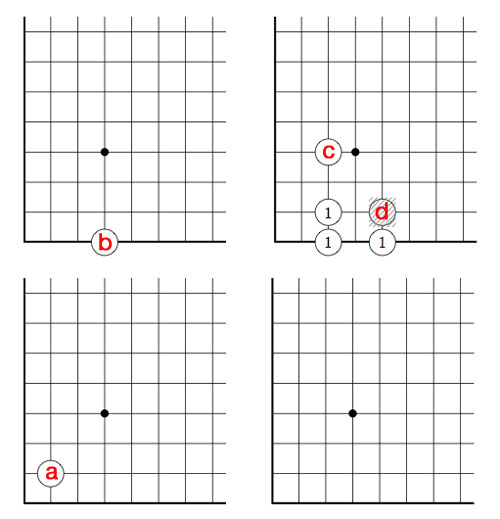Above is the unique winning move : we remove stone d and by the rectangle-rule add three new stones, marked 1. We only need to compute in finite fields to solve this and similar problems.

First note that the largest finite number occuring in a stone-coordinate is 4, so in this case we can perform all calculations in the field $\mathbb{F}_{2^{2^2}}(\omega) = \mathbb{F}_{2^{12}}$ where (as before) we identify $\mathbb{F}_{2^{2^2}} = { 0,1,2,\ldots,15 }$ and we have seen that $\omega^3=2$ (for ease of notation all Nim-additions and Nim-multiplications are denoted this time by + and x instead of $\oplus$ and $\otimes$ as we did last time, so for example $\omega^3 = \omega \otimes \omega \otimes \omega$).

If you’re not nimble with the Nim-tables, you can check all calculations in SAGE where we define this finite field via

sage: R.< x,y,z>=GF(2)[]
sage: S.< t,f,o>=R.quotient((x^2+x+1,y^2+y+x,z^3+x))

and we can now calculate in $\mathbb{F}_{2^{12}}$ using the symbols t for Two, f for Four and o for $\omega$. For example, we have seen that the nim-value of a stone is the nim-multiplication of its coordinates

sage: t*t
t + 1
sage: f*o
f*o
sage: (o+t)*(o+t+1)
o^2 + o + 1
sage: (o+f)*(o+1)
f*o + o^2 + f + o

That is, the nim-value of stone a is 3, stone b is $4 \times \omega$, stone c is $\omega^2+\omega+1$ and finally that of stone d is equal to $\omega^2+5 \times \omega +4$.

By adding them up, the nim-value of the original position is a finite number : 6. Being non-zero we know that the 2nd player has a winning strategy.

Just as in ordinary nim, we compare the value of a stone to the sum of the values of the other stones, to determine the stone we will play. These sums are for the four stones : 5 for a, $4 \times \omega+6$ for b, $\omega^2+\omega+7$ for c and $\omega^2+5 \times \omega+2$ for d. There is only one stone where this sum is smaller than the stone-value, so we know we have to make our move with stone d.

By the Nimbers-rule we need to find a rectangle with top-right hand corner $~(\omega+4,\omega+1)$ and lower-left hand corner $~(u,v)$ such that the values of the three new corners adds up to $\omega^2+5 \times \omega+2$, that is we have to solve

$u \times v + u \times (\omega+1) + (\omega+4)\times v = \omega^2+5 \times \omega+2$

where u and v are ordinals smaller than $\omega+4$ and $\omega+1$. u and v cannot be both finite, for then we wouldn’t obtain a $\omega^2$ term. Similarly (u,v) cannot be of the form $~(u,\omega)$ with u finite because then the left-hand sum would be $\omega^2+4 \times \omega + u$ and likewise it cannot be of the form $~(\omega+i,v)$ with i and v finite as then the coefficient of $\omega$ in the left-hand sum will be i+1 and we cannot take i equal to 4.

The only remaining possibility is that (u,v) is of the form $~(\omega+i,\omega)$ with i finite, in which case the left-hand sum equals $~\omega^2+ 5 \times \omega + i$ whence i=2 and we have found our unique winning move!

But, our opponent can make life difficult by forcing us to compute in larger and larger finite fields. For example, if she would move next by dropping the c stone down to the 256-th row, what would be our next move?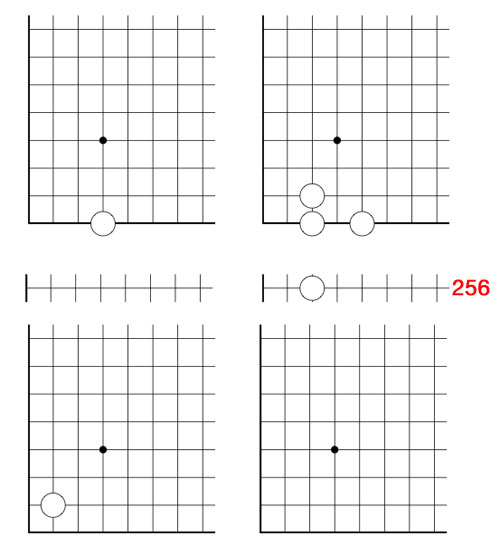(one possible winning move is to remove the stone at $~(\omega+2,\omega)$ and add stones at the three new corners of the rectangle : $~(257,2), (257,\omega)$ and $~(\omega+2,2)$)

Today, we will expand the game of Nimbers to higher dimensions and do some transfinite Nimber hacking.

In our identification between $\mathbb{F}_{16}^*$ and 15-th roots of unity, the number 8 corresponds to $\mu^6$, whence $\sqrt{8}=\mu^3=14$. So, if we add a stone at the diagonal position (14,14) to the Nimbers-position of last time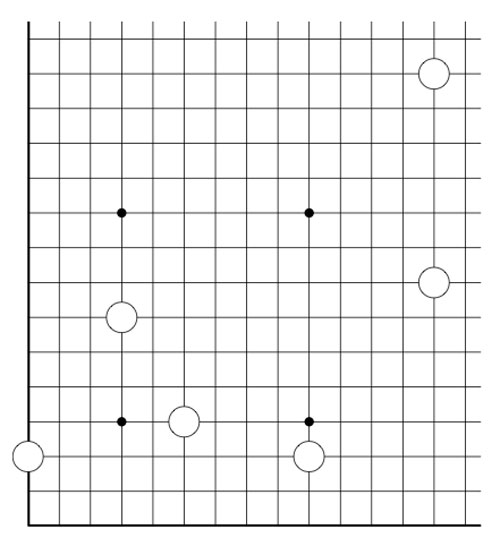we get a position of Nim-value 0, that is, winnable for the second player. In fact, this is a universal Nimbers-truth :

Either the 2nd player wins a Nimbers-position, or one can add one stone to the diagonal such that it becomes a 2nd player win.

The proof is elementary : choose a Fermat 2-power such that all stones have coordinates smaller than $2^{2^n}$. If the Nim-value of the position isn’t zero, it corresponds to a unit $\alpha \in \mathbb{F}_{2^{2^n}}^*$. Now,the Frobenius map $x \mapsto x^2$ is an automorphism of any finite field of characteristic two, so the square root $\sqrt{\alpha}$ also belongs to $\mathbb{F}_{2^{2^n}}$, done!

3-dimensional Nimbers is played in the first octant of the integral lattice $\mathbb{Z}^3$ by placing a finite number of balls at places $~(a,b,c) \in \mathbb{N}^3$ with $abc \geq 1$.

Moves are defined by replacing the rectangular-rule of the two-dimensional version by the cuboid-rule : take a cuboid with faces parallel to the coordinate planes whose corner of maximal distance from the origin is one of the balls in the position. Remove that ball and add new balls to the unoccupied corners and remove balls at occupied corners.

Here, we allow the corner-points to have zero as some of its coordinates, but these balls are considered dead in the game. As in the two-dimensional game, this cuboid-rule encompasses several legal moves depending on the number of corners in the cuboid having zero-coordinates.

Again, it follows by induction that the Nim-value of a ball placed at position $~(a,b,c)$ is equal to the Nim-multiplication $a \otimes b \otimes c$ and we can calculate the Nim-value of a 3-dimensional Nimbers-position by computing in a suitable field $\mathbb{F}_{2^{2^n}}$. (The extension to higher dimensions is now obvious)

Does 3-dimensional Nimbers satisfy the ‘universal truth’, that is, can one make any position a 2nd player win by adding at most one stone to the body-diagonal?

The previous argument fails. As $\mathbb{F}_4^*$ is the cyclic group of order three, the 3rd roots of unity in $\overline{\mathbb{F}_2}$ correspond to the numbers 1,2 and 3, so the map $x \mapsto x^3$ cannot be a bijection on any of the finite fields $\mathbb{F}_{2^{2^n}}$.

But then, perhaps, a third root is added by going to a larger such field $\mathbb{F}_{2^{2^N}}$? Well, not quite. Take for example 2, then $\sqrt{2} \notin \mathbb{F}_{2^{2^N}}$. (2 has order 3 in $\mathbb{F}_4$ and so its 3rd root must have order 9, but 9 does not divide any number of the form $2^{2^N}-1$ as the Fermat-powers mod 9 can only be 4 or 7).

In fact one can show that this also holds for any number not in the image of the cubing-map in some $\mathbb{F}_{2^{2^n}}$ as

$\mathbb{N}={ 0,1,2,\ldots } = \cup_N \mathbb{F}_{2^{2^N}}$

with Nim-addition and multiplication is the quadratic closure of $\mathbb{F}_2$ (see for example ONAG).

The situation changes if we allow ourself to play transfinite Nimbers, with the same rules as before but now we allow the stone, balls etc. to be placed at points of which the coordinates are not restricted to $\mathbb{N}_+ = \{ 1,2,3,\ldots \}$ but may vary over $[\beta]_+$ for some ordinal $\beta$ where $[\beta]_+=\{ 1,2,\ldots,\omega,\omega+1,\ldots \}$ is the set of all ordinals smaller than $\beta$.

In transfinite 3-dimensional Nimbers the ‘universal truth’ still holds, provided we play it on a cube of sizes $[\omega^{\omega}]_+$. In particular we have that $\sqrt{2}=\omega$ by the simplicity rule (see ONAG or the Conway’s nim-arithmetic post)

In general, n-dimensional transfinite Nimbers played on an n-gid of sizes $[\omega^{\omega^{\omega}}]_+$ satisfies the universal truth : either a position is a 2nd player win or it becomes one by adding one n-ball to a diagonal position! (this follows immediately because $[\omega^{\omega^{\omega}}]$ with Nim-addition and multiplication is isomorphic to the algebraic closure of $\mathbb{F}_2$).

2-dimensional transfinite Nimbers is still pretty playable. Below a position on a $\omega.2$-board with stones as positions $~(2,2),(4,\omega),(\omega+2,\omega+3)$ and $~(\omega+4,\omega+1)$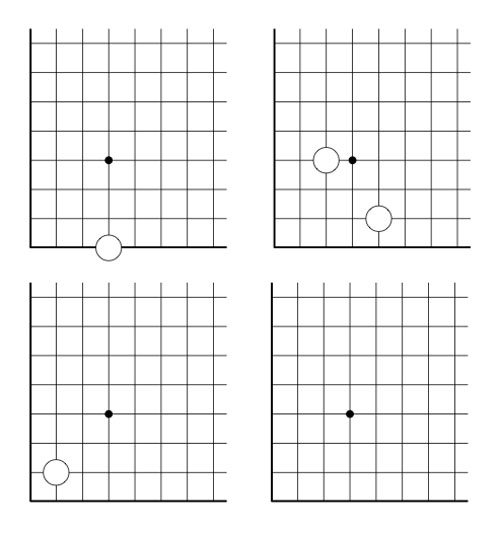Give a winning move for the first player!